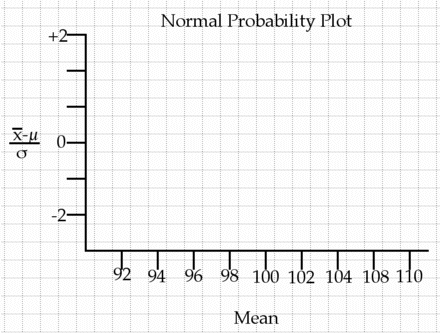# Sampling from the Normal Distribution

This handy little page will simulate any Normal Distribition, so you can notice certain trends in certain statistics when one goes around and takes a sample from a larger, normally distributed population.
 Population mean (mu): Population standard deviation (sigma): Sample size (n):

## Instructions

1. Simulate making 25 observations from a population whose mean is 100 and standard deviation is 15 (i.e. N[100,15])

2. Compare the sample mean (x-bar) to the population mean (mu).

3. Compare the sample std. dev. (s) to the population std. dev (sigma).

4. Begin building a table with three headings: "Sample Number", "Sample Mean", and "Sample Std. Dev." and record your first simulation.

5. Repeat the procedure until you have simulated taking a sample of 25 observations 20 times.

6. Now make a stem plot of the 20 means.

7. Is it skewed or semetric? Where is its center?

8. What is the mean of the 20 means? Is it different from the population mean?

9. What is the std. dev of the 20 means? Is is different from the population std. deviation?

10. Compute the population std. dev. (sigma) divided by the square root of the sample size (n=25)

11. How does this compare with the the standard deviation of the 20 means?

12. Make find the "z-score" of each of the 20 sample means to make a Normal Probability Plot. Is the plot linear? What does this imply?13. Make a prediction about simulating taking a sample of 16 observations from a population normally distributed N[50,12]. What would the sample mean look like? The sample std. dev?

14. Have the computer simulate this...how does it compare with your prediction?

15. Do this 19 more times (Make a new table). What do you think the mean of the means will be? What do you think the standard deviation of the means will be?

16. How did your prediction compare with your 20 simulations of sampling an N[50,12] with sample size n=16?